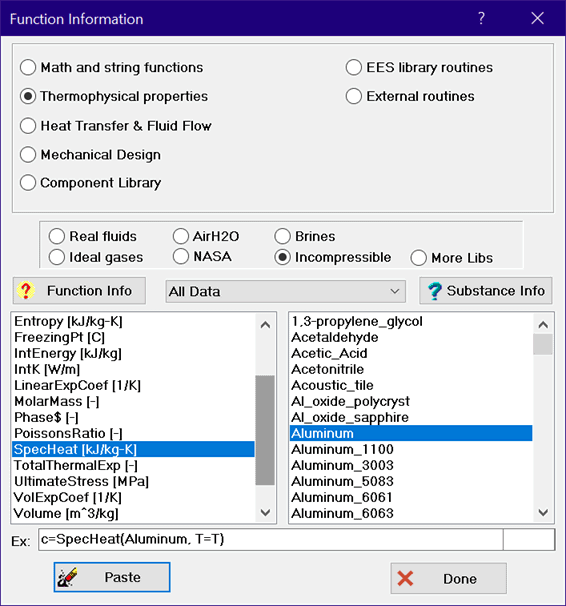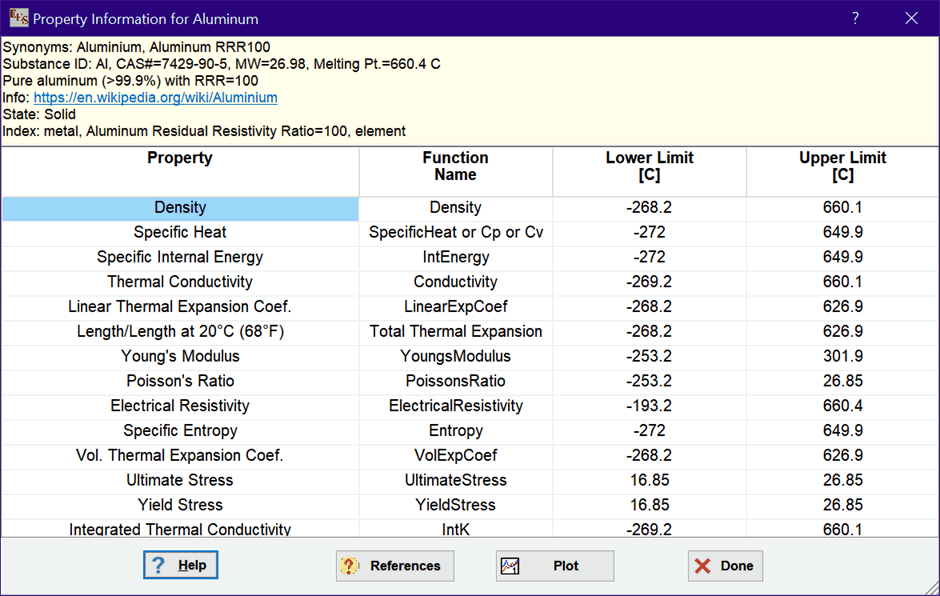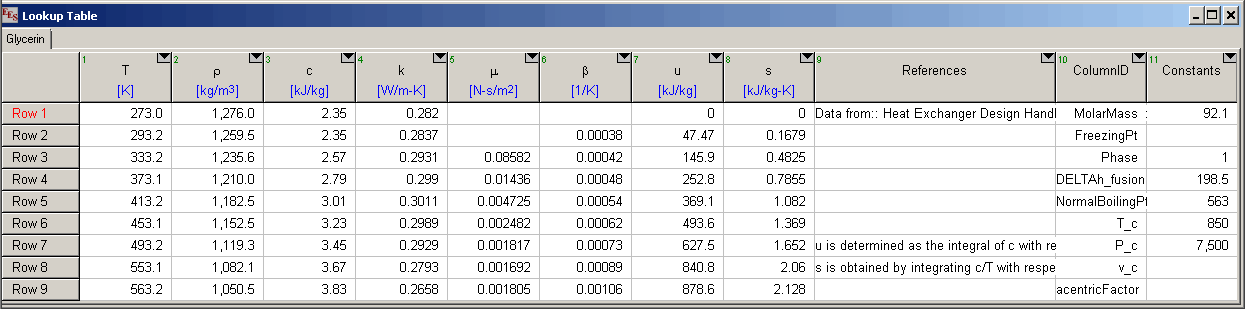Contents - Index

Incompressible Substances

Clicking the Thermophysical Properties button in the Function Information dialog will configure the Function Information dialog to appears as shown.The list on the left shows all of the property functions that are implemented for incompressible solid and liquid substances.  However, not all functions are available for all substances.   Click the Function Info button at the top of the list for information relating to the selected function.

The list on the right are names of substances to which these functions can be applied.  The drop-down list box in the center allows a selection criterion to be applied to the list of substances.  The available selection criteria are:  All data, metals, liquid metals, building materials, heat transfer fluids, insulation, molten salts, organic liquids, and miscellaneous.  Some substances may appear in more than one list.  Clicking the Property Info button will provide a summary of the property information available for the selected substance, as shown here for copper.Click the References button to access the source of the property data and other information.

An example indicating the format of the function use appears in the examples box.  Most of the property functions require only temperature as an input.  Note that, for consistency with the properties of real and ideal gas substances, the temperature is preceded with the T= identifier.  The Temperature can be a numerical value, and EES variable, or an algebraic expression that evaluates to the desired temperature in the scale selected in the Unit System dialog.  For example, the following equations provides the thermal conductivity of aluminum at 300 K

\$UnitSystem SI K kPa kJ mass

T=300 [K]

k=Conductivity('Aluminum', T=T)

Enthalpy requires pressure as well as temperature.  In this case, the P= indicator must be supplied to the left of the pressure. The temperature and pressure can appear in any order, e.g.,

h=enthalpy('Aluminum', T=T, P=P)

Data for incompressible substances are provided in EES Lookup tables having an .LKT filename extension.  Shown below is the table for GlycerinThe data for a particular substance can be modified or extended by the user.  The tables reside in the USERLIB\EES_SYSTEM\INCOMPRESSIBLE folder within the folder that EES is installed.  Data for additional substances can also be added by supplying a new Lookup table for each substance.  Each temperature-dependent property is provided in a separate column of the table.  Note that only the following properties are recognized and the data must be entered in the specified units.  These data are temperature dependent.  Temperature should be the first column in the Lookup table.  The names of the columns and their corresponding function names are as follows.

Column Name Function Name Property Units

alpha LinearExpCoef linear coef. of thermal expansion 1/K

beta VolExpCoef volumetric coef. of thermal expansion 1/K

c SpecHeat (or Cp or Cv) specific heat capacity kJ/kg-K

DELTAh_vap Enthalpy_vaporization specific enthalpy change of vaporization kJ/kg

DELTAL\L_293 TotalThermalExp linear expansion1 none

E YoungsModulus Young's modulus GPa

epsilon Emissivity emissivity none

k Conductivity thermal conductivity W/m-K

mu Viscosity dynamic viscosity Pa-s

nu PoissonsRatio Poisson's ratio none

Pv P_sat vapor pressure kPa

rho Density density kg/m3

rho_e ElectricalResistivity electrical resistivity ohm-m

s Entropy specific entropy kJ/kg-K

sigma SurfaceTension surface tension N/m

Su UltimateStress ultimate stress MPa

Sy YieldStress yield stress MPa

u IntEnergy specific internal energy kJ/kg

The volumetric coefficient of thermal expansion (beta)  is commonly used in thermal problems whereas the linear coefficient of thermal expansion (alpha) is commonly used in mechanics problems.  The two coefficients are related by beta=3*alpha is the substance is isotropic.

The density variation with temperature of most substance can be calculated from the density at a known temperature (usually 293 K) and DELTAL\L_293, the change in length relative to the length at 293K (20�C or 68�F).  For example, the density of copper is known at 300 K to be 8933 kg/m^3.  Then, then the density at a  temperature of 800 K can be found from the value of TotalThermExp function (DELTAL\L_293) at 800K from:

rho_300=8933  [kg/m^3]

DELTAL\L_293=0.00939

rho_T=rho_300/(1+DELTAL\L_293)^3

The elastic modulus, E and the shear modulus, G, are related through Poisson's ratio, n, by E/G=2*(1+n).  Note that n here is Poisson's ratio and not the kinematic viscosity.

If a column is provided for specific internal energy, the value of is determined as the integral of specific heat with respect to T, with the zero value occurring at the lowest temperature for which data for specific heat data are provided.  The specific enthalpy is found using u + P/rho.  Note that both T and P are required as inputs to the specific enthalpy function. If a column  is provided for specific entropy, it has been determined as the integral of specific heat divided by temperature, integrated with respect to temperature.

Reference information for each column of data is provided in the corresponding row of Reference column.  Note that this column must be configured for string data.  The first row in the References column provides general information.  Subsequent rows provide reference information for the data that reside in the column with the same position as the row.  In the table shown above, information provided in the second row of the References column would be applicable to rho (density), which resides in the second column in the table.  The reference data are optional, but recommended  String information provided for rows numbers greater than the number of data columns are also assumed to provide general information, which is displayed when the Reference button is clicked.  Each string can consist of up to 255 characters.

The ColumnID column also contains string data and it identifies the constants in the last column.  The order of these constants must appear as shown.  If a property value is not known, leave it blank.

Molar Mass

Freezing Point (K)

Phase (0 for solid, 1 for liquid)

DELTAh_fusion (kJ/kg)

Normal Boiling Pt (K)

Critical temperature (K)

Critical pressure (kPa)

Critical volume (m^3/kg)

Acentric factor

Note:  If you change or add an Incompressible substance table, make a copy and put it in a directory other than the EES directory to ensure that it will not be overwritten when you next update EES.

Note that the Incompressible library replaces the Solid-Liquid property library provided in versions prior to version 9.275.  However, all of the functions in the Solid-Liquid library are supported for backward compatibility.